# Distortion and harmonics

Submitted by mic on Wed, 03/28/2018 - 21:12

admin: First posted on 2011 09 03

Guest post by Anton Kamenov

Distortion in music compresses the peaks of the audio signal. In the analog world this can happen when the signal begins to overload internal circuits. Digital distortion is similar.

Take the following simple computation of the output signal y(k) from the input signal x(k).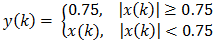This operation is known as a hard clip. It simply cuts off the peaks of a simple sine wave with peak amplitude of 1 as in the figure below. The figure below shows a wave with frequency of 100 Hz and peak amplitude of 1, sampled at 2000 Hz. The wave is truncated with the formula above.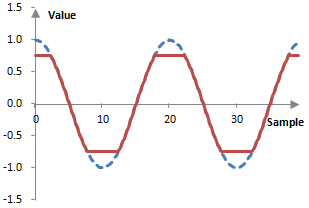Hard-clipping with introduce distortion, but this is not the end of the story. The resulting wave is no longer just a simple wave. It is some signal with some frequency content that is different than the frequency content of the original wave.

The figure below shows the frequency content of the original simple wave and of the distorted signal. While the input simple wave has only one peak at 100 Hz – the frequency of the simple wave – the distorted signal has additional notches at 300 Hz, 500 Hz, and 700 Hz.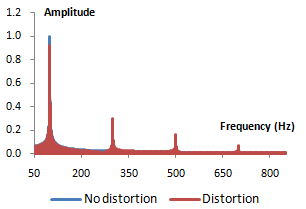These frequencies are odd integer multiples of 100 Hz and are thus odd order harmonics of 100 Hz. Thus, distortion introduces harmonics. Any nonlinear modification of the signal would in fact introduce harmonics. A nonlinear modification to the signal can thus be called "harmonic distortion". The more severe the clipping is (e.g., 0.5 instead of 0.7), the more accentuated the harmonics will be.

A sharp truncation of the signal peaks is not the only way to introduce distortion. A softer distortion can be introduced by slowly compressing and flattening of the peaks. The cubic soft clipper is a well known function that does exactly that.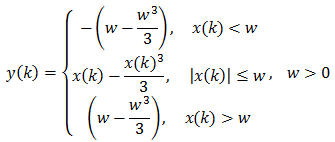The impact of the cubic soft clipper on a simple wave is shown below. With larger w, the clipping becomes softer and closer to the peak of the wave. The cubic soft clipper introduces similar odd order harmonics in the signal.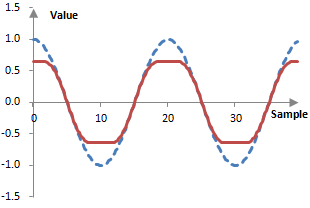There are many operations that we can use to clip the signal similarly. Clipping need not always occur. The following distortion operation compresses the signal peaks without actually clipping them. It introduces the same odd order harmonics, although with lower energy.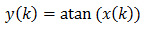It is interesting that clipping and compressing the peaks of the wave always introduces odd order harmonics. Consider the following figure, which shows a signal at 100 Hz with peak amplitude of 1 (dashed blue) and the sum of the signal at 100 Hz with a signal at 300 Hz with an amplitude of 0.3 (red).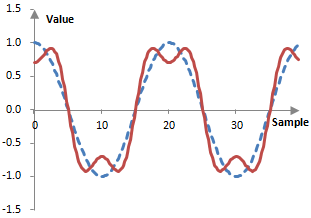The impact of adding a simple wave with three times the frequency and lower amplitude is very similar to the impact of clipping the wave. Thus, odd order harmonics during distortion are unavoidable.

If we wanted to introduce even order harmonics – even integer multiples of the original frequency – we could examine the impact of adding two waves, one of which is, say, twice the frequency of the other one – the first even order harmonic. The sum of two such waves is shown below.Even order harmonics do not compress, but expand the signal peaks and troughs. Further, the signal becomes asymmetric – unlike adding the first odd order harmonic, which produces a rather symmetric signal.

One modification that is a good candidate for introducing even order harmonics is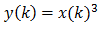You can verify that this particular function introduces both even and odd order harmonics. Clipping this signal would produce a very different distortion.

The following modification could also be of interest.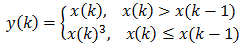This operation introduces only even order harmonics, except for the first odd order harmonic at three times the original frequency, as below.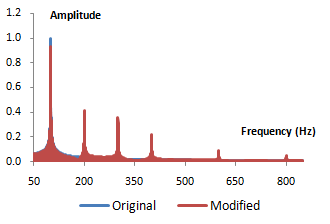The only issue with this operation is that it is not memoryless. It has to look back at previous samples of the signal.

Digital distortion in practice could be as simple as the operations above or more complex. For example, distortion could require upsampling of the audio data. This may be necessary, because harmonics of high recorded frequencies may be higher than the Nyquist-Shannon frequency for the original sampling rate. Upsampling recreates the signal, but at a higher sampling rate, at which the Nyquist-Shannon frequency would also be higher. Digital distortion may also include an equalizer, so that the magnitude of harmonics is controlled. Finally, distortion may distort different parts of the frequency spectrum differently, by splitting the original signal into several parts, say, low, middle, and high frequencies.

### Filtered HTML

• Freelinking helps you easily create HTML links. Links take the form of [[indicator:target|Title]]. By default (no indicator): Click to view a local node.